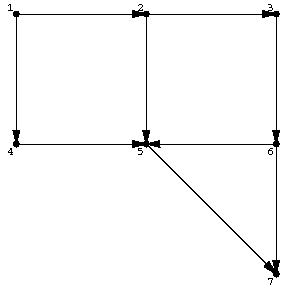# Search algorithm

on a graph, graph search

An algorithm that tries to find all nodes in a network or graph that satisfy a given property. Such algorithms are also used, e.g., to optimize a function on a graph or network or on a set which can easily be turned into a graph, such as $\{ 0,1 \} ^ {n}$. (Two $0$- $1$ vectors of length $n$ are joined if an only if they differ in precisely one coordinate, yielding a hypercube.) Thus, these are definite relations and similarities between graph search and search theory as a systematic optimization technique (of enumeration type) [a3]. See [a4] for a very complete overview of global optimization (from the search point of view, including random search).

Two of the most often used search techniques in graphs or networks are depth-first search and breadth-first search. Let $\Gamma = ( V,E )$ be a finite oriented graph (cf. Graph, oriented). For each $v \in V$, let $A ( v )$ be the set of edges (arcs) issuing from $v$. For instance, in Fig.a1, $A ( 1 ) = \{ ( 1,2 ) , ( 1,4 ) \}$, $A ( 3 ) = \{ ( 3,6 ) \}$, $A ( 7 ) = \emptyset$.Figure: s110070a

It is assumed that the sets $A ( v )$, $v \in V$, are given some fixed total order (cf. also Totally ordered set) for all $v$. The following algorithm picks out all nodes in $\Gamma$ that are reachable from a source node $s$ by means of a directed path. These nodes will be marked. Central to the algorithm is a certain list $L$, which can be seen as a sort of wave front indicating the boundary of the spreading blob of marked points:

unmark all points;

mark the source node $s$;

set $L = \{ s \}$;

as long as $L$ is non-empty, run the following iterative procedure:

choose a node $i \in L$;

select the first oriented edge $( i,j ) \in A ( i )$ that runs (from $i$) to an unmarked node $j$( if any);

mark the node $j$;

define the function ${ \mathop{\rm revp} }$ on $j$ as ${ \mathop{\rm revp} } ( j ) = i$;

add $j$ to $L$;

if there is no $( i,j ) \in A ( i )$ that runs to an unmarked node $j$, remove $i$ from $L$.

stop when $L$ is empty.

The algorithm does not yet specify how to select a node from $L$. If $L$ is treated as a queue, i.e., the node selected is the one that has been longest in $L$( first-in-first-out) the result is breadth-first search. On the other hand, if $L$ is treated as a stack, i.e., the node selected is the one that was last added to $L$( last-in-first-out) the result is depth-first search.Figure: s110070b

For an oriented tree as in Fig.a2 (downwards oriented), starting at the indicated node, breadth-first search will first pick the three children of the node indicated (in some order) and then will proceed to the next layer. Depth-first search will first pick a maximal downward chain (maximal in the sense that it ends at a leaf, not necessarily maximal in length) and then go back to another child of the last branching node in that chain.

The order which is used on the edge sets $A ( v )$ also matters, but not as much.

The edges picked out by the function ${ \mathop{\rm revp} }$( reverse path), i.e., the edges $( { \mathop{\rm revp} } ( j ) ,j )$ form an oriented tree comprising the reachable nodes from the source node $s$.

The results of the algorithm in four cases are given below for the case of Fig.a1.

<tbody> </tbody>
 search marking oriented method sequence tree breadth first, 1 2 4 3 5 6 7lexicographic order on the $A ( i )$ breadth first, 1 4 2 5 3 7 6reverse lexicographic order on the $A ( i )$ depth first 1 2 3 6 5 7 4lexicographic order on the $A ( i )$ depth first 1 4 5 7 2 3 6reverse lexicographic order on the $A ( i )$

There is an obvious analogous search algorithm for non-oriented graphs. These algorithms run in $O ( \# V + \# E )$ time.

How to Cite This Entry:
Search algorithm. Encyclopedia of Mathematics. URL: http://encyclopediaofmath.org/index.php?title=Search_algorithm&oldid=48636
This article was adapted from an original article by M. Hazewinkel (originator), which appeared in Encyclopedia of Mathematics - ISBN 1402006098. See original article